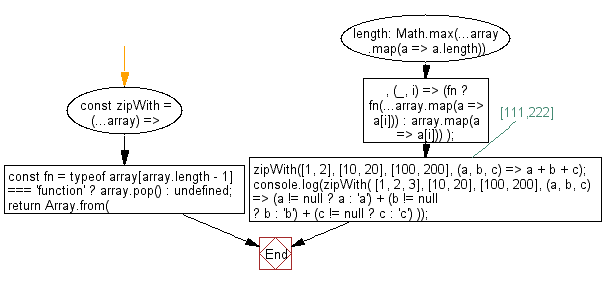# JavaScript : Create an array of elements, grouped based on the position in the original arrays and using function as the last value to specify how grouped values should be combined

## JavaScript fundamental (ES6 Syntax): Exercise-100 with Solution

Write a JavaScript program to create an array of elements, grouped based on the position in the original arrays. Use function as the last value to specify how grouped values should be combined.

Note: Check if the last argument provided is a function.

• Check if the last argument provided is a function.
• Use Math.max() to get the longest array in the arguments.
• Use Array.from() to create an array with appropriate length and a mapping function to create array of grouped elements.
• If lengths of the argument arrays vary, undefined is used where no value could be found.
• The function is invoked with the elements of each group.

Sample Solution:

JavaScript Code:

``````//#Source https://bit.ly/2neWfJ2
const zipWith = (...array) => {
const fn = typeof array[array.length - 1] === 'function' ? array.pop() : undefined;
return Array.from(
{ length: Math.max(...array.map(a => a.length)) },
(_, i) => (fn ? fn(...array.map(a => a[i])) : array.map(a => a[i]))
);
};

zipWith([1, 2], [10, 20], [100, 200], (a, b, c) => a + b + c); // [111,222]

console.log(zipWith(
[1, 2, 3],
[10, 20],
[100, 200],
(a, b, c) => (a != null ? a : 'a') + (b != null ? b : 'b') + (c != null ? c : 'c')
));
```
```

Sample Output:

```[111,222,"3bc"]
```

Flowchart:Live Demo:

See the Pen javascript-basic-exercise-100-1 by w3resource (@w3resource) on CodePen.

Improve this sample solution and post your code through Disqus

What is the difficulty level of this exercise?

Test your Programming skills with w3resource's quiz.

﻿